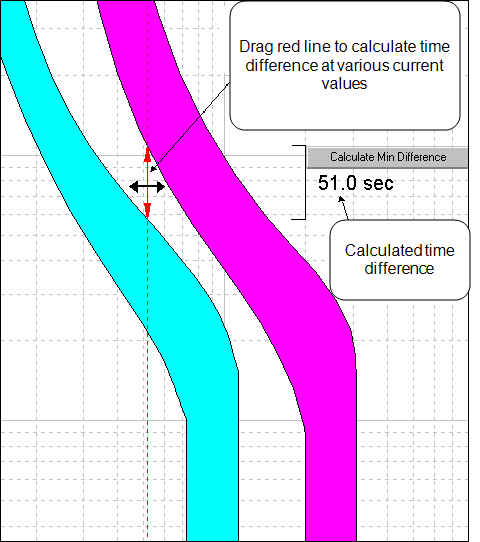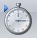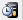# Insert Difference CalculatorFigure 1: Using Difference Calculator

Using the Difference Calculator, you can calculate the difference in trip time between the TCC plot of any two protective devices at any given current value, as follows:

1. Select any two TCC curves on the TCC plot. To select two curves, hold down SHIFT and select the two curves in the TCC window.
2. ClickDifference Calc (Insert Time Difference Calculator) in the TCC tab. When you insert a difference calculator, a red vertical line appears representing a current value as shown in the figure above. Next to it, the calculated time difference appears along with a time difference marker. The time difference marker is a line that looks like a square bracket (]).
3. Drag the red line to calculate the time difference at various current values.

To calculate the minimum time difference between the two curves:

1. Select the time difference marker and click the arrow belowDifference Calc.
2. ClickCalculate Min Difference. The red line moves to the current value at which the minimum time difference occurs between the two curves.

Note: You can also right-click after selecting the time difference marker and click Calculate Min Difference from the menu.